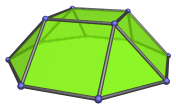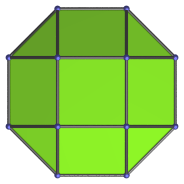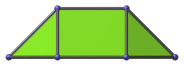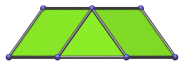# The Square Cupola

The square cupola is one of the Johnson solids. It is bounded by 4 equilateral triangles, 5 squares, and an octagon, for a total of 10 faces, 20 edges, and 12 vertices. It is listed as the fourth polyhedron in Norman Johnson's list, and thus bears the label J4.The square cupola is related to the square pyramid (J1). Starting with the square pyramid, if we push out the triangular faces radially along the plane of its base while retaining their respective orientations, the apex of the pyramid will break apart into 4 vertices, which can be connected by inserting a square. The vertices of the base will also break apart into 8 vertices, and when the distance between vertices are equal to the original edge length, we can fill in the resulting gaps with squares and an octagon to make a square cupola. Thus, we may think of the square cupola as an “expanded” square pyramid.

The square cupola also occurs as a segment of the rhombicuboctahedron, which may be thought of as an elongated square orthobicupola: the result of pasting together a square cupola, an octagonal prism, and a second square cupola. Thus, the square cupola may be thought of as “one third” of a rhombicuboctahedron (though strictly speaking, it is not exactly one third). If the two square cupolae are not aligned with each other, this pasting results in the elongated square gyrobicupola (J37) instead.

Pasting only one square cupola with an octagonal prism produces the elongated square cupola (J19). Pasting it with an octagonal antiprism instead produces the gyroelongated square cupola (J23), which can be extended by adding a second cupola to produce the gyroelongated square bicupola (J45). Pasting two square cupolae together without any intervening prism produces the square orthobicupola (J28) or the square gyrobicupola (J29), depending on the orientation.

The square cupola can also augment a truncated cube to produce the augmented truncated cube (J66), one of the Johnson solids. Adding a second square cupola on the opposite side produces the biaugmented truncated cube (J67).

## Projections

In order to be able to identify the square cupola in various projections of 4D objects, it is useful to know how it appears from various viewpoints. The following are some of the viewpoints that are commonly encountered:

Projection Envelope DescriptionRegular octagon

Parallel projection from the top.Trapezium

Parallel projection from the side, centered on a square face.Trapezium

Parallel projection from the side, centered on a triangular face.

## Coordinates

The coordinates of the square cupola may be easily derived from its relationship with the rhombicuboctahedron. Starting with the coordinates of the latter as all permutations of coordinate and changes of sign of (±1, ±1, ±(1+√2)), we simply omit the points whose last coordinate is negative. We thus obtain:

• (±1, ±1, 1+√2)
• (±1, ±(1+√2), 1)
• (±(1+√2), ±1, 1)

These coordinates give us a square cupola of edge length 2; however, the cupola is not centered on the origin. A little algebra shows that we simply need to subtract (3+√2)/3 from the last coordinates, and so we obtain the following origin-centered coordinates:

• (±1, ±1, (2/3)√2)
• (±1, ±(1+√2), −(1/3)√2)
• (±(1+√2), ±1, −(1/3)√2)

## Occurrences

The square cupola occurs as cells in a number of 4D CRF polychora, including (but by no means limited to) the following:

Last updated 18 Jun 2019.